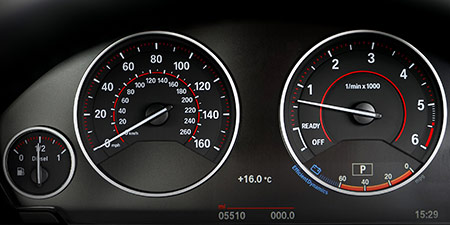# Speed of light to Miles per hour (mph)

Thema Units of speed ﹣ Converter Speed of light to Miles per hour

Here you can convert the Speed unit Speed of light into the unit Miles per hour and vice versa you can convert Miles per hour into Speed of light. By clicking the "Swap units" icon, you will always obtain the desired conversion in the calculation result. With the following calculator you can also calculate any other Speed unit.

## Info about "Speed of light"

Definition: If an object moves at the light speed for one second, it travels a distance of 299,792.458 km or about 300,000 km. This is the light speed that light travels in a vacuum.

This speed is also used to propagate radio signals, which is very important in space travel. For example, a signal from Mars takes about 3 to 20 minutes to reach Earth depending on its actual position. We notice a sunrise on earth about 8 minutes later because the light of the sun arrives to our eyes at that time.

## Info about "Miles per hour"

The unit of length known as a "mile" and it should not be confused with the nautical mile and very commonly used in the Anglo-American countries. Therefore, the metric system is defined in miles (instead of kilometers) per time unit. For example, if a vehicle travels at a speed of 100 miles per hour (100 mph), it will move around 160 km per hour in the metric system.

The units are defined as follows: An object moving at 1 "mph" per hour will cover a distance of one mile. Therefore, 1 mile is equivalent to 1.609344 kilometers.

## Formula for the conversion of Speed of light to Miles per hour (mph) and vice versa

The calculation from Speed of light to Miles per hour shall be made using the following conversion formula:

Conversion formula Speed of light to Miles per hour

Determine the number of Miles per hour from Speed of light

Speed of light × 670616611.75872

## Formula for the conversion of Miles per hour (mph) to Speed of light

The calculation from Miles per hour to Speed of light shall be made using the following conversion formula:

Conversion formula Miles per hour to Speed of light

Determine the number of Speed of light from Miles per hour

Miles per hour × 1.4911649703658E-9

## Overview table : How many Speed of light are in Miles per hour ?

Speed of light ⇒ Miles per hour mph
0.01   are  6 706 166.11758 mph
0.02   are  13 412 332.23517 mph
0.03   are  20 118 498.35276 mph
0.04   are  26 824 664.47034 mph
0.05   are  33 530 830.58793 mph
0.06   are  40 236 996.70552 mph
0.07   are  46 943 162.82311 mph
0.08   are  53 649 328.94069 mph
0.09   are  60 355 495.05828 mph
0.10   are  67 061 661.17587 mph
0.20   are  134 123 322.35174 mph
0.30   are  201 184 983.52761 mph
0.40   are  268 246 644.70348 mph
0.50   are  335 308 305.87935 mph
0.60   are  402 369 967.05523 mph
0.70   are  469 431 628.23110 mph
0.80   are  536 493 289.40697 mph
0.90   are  603 554 950.58284 mph
1   corresponds to  670 616 611.75871 mph
2   are  1 341 233 223.51743 mph
3   are  2 011 849 835.27615 mph
4   are  2 682 466 447.03486 mph
5   are  3 353 083 058.79358 mph
6   are  4 023 699 670.55230 mph
7   are  4 694 316 282.31102 mph
8   are  5 364 932 894.06973 mph
9   are  6 035 549 505.82845 mph
10   are  6 706 166 117.58717 mph
20   are  13 412 332 235.17434 mph
30   are  20 118 498 352.76152 mph
40   are  26 824 664 470.34869 mph
50   are  33 530 830 587.93586 mph
60   are  40 236 996 705.52304 mph
70   are  46 943 162 823.11021 mph
80   are  53 649 328 940.69738 mph
90   are  60 355 495 058.28455 mph
100   are  67 061 661 175.87172 mph
200   are  134 123 322 351.74345 mph
300   are  201 184 983 527.61520 mph
400   are  268 246 644 703.48690 mph
500   are  335 308 305 879.35864 mph
600   are  402 369 967 055.23040 mph
700   are  469 431 628 231.10211 mph
800   are  536 493 289 406.97381 mph
900   are  603 554 950 582.84558 mph
1 000   are  670 616 611 758.71728 mph

## Overview table : How many Miles per hour are in Speed of light ?

Miles per hour mph ⇒ Speed of light
0.07 mph  are  0.0000000001
0.08 mph  are  0.0000000001
0.09 mph  are  0.0000000001
0.10 mph  are  0.0000000001
0.20 mph  are  0.0000000003
0.30 mph  are  0.0000000004
0.40 mph  are  0.0000000006
0.50 mph  are  0.0000000007
0.60 mph  are  0.0000000008
0.70 mph  are  0.0000000010
0.80 mph  are  0.0000000011
0.90 mph  are  0.0000000013
1 mph  corresponds to  0.0000000014
2 mph  are  0.0000000029
3 mph  are  0.0000000044
4 mph  are  0.0000000059
5 mph  are  0.0000000074
6 mph  are  0.0000000089
7 mph  are  0.0000000104
8 mph  are  0.0000000119
9 mph  are  0.0000000134
10 mph  are  0.0000000149
20 mph  are  0.0000000298
30 mph  are  0.0000000447
40 mph  are  0.0000000596
50 mph  are  0.0000000745
60 mph  are  0.0000000894
70 mph  are  0.0000001043
80 mph  are  0.0000001192
90 mph  are  0.0000001342
100 mph  are  0.0000001491
200 mph  are  0.0000002982
300 mph  are  0.0000004473
400 mph  are  0.0000005964
500 mph  are  0.0000007455
600 mph  are  0.0000008947
700 mph  are  0.0000010438
800 mph  are  0.0000011929
900 mph  are  0.0000013420
1 000 mph  are  0.0000014911

## Source information

As source for the information in the "Units of speed" category, we have used in particular:

## Last update on May 3, 2022

The pages of the "Units of speed" category were last editorially reviewed by Stefan Banse on May 3, 2022. They all correspond to the current status.

### Previous changes on October 9, 2021

• November 9, 2020: Publication of the Speed converter
• Editorial revision of all texts in this category